true

Sumita D.

Hauz Khas, Delhi, India - 110016# Sumita D.

## Tutor

Hauz Khas, Delhi, India - 110016.

13 Students taught

Referral Discount: Get ₹ 500 off when you make a payment to start classes. Get started by Booking a Demo.

Details verified of Sumita D.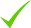IdentityEducation

Know how UrbanPro verifies Tutor details

Identity is verified based on matching the details uploaded by the Tutor with government databases.

## Overview

I am taking home tuition for last 8 yrs. I have a capability to work harder and i know how to manage a student, how to deal with them and i have a good teaching skills and knowledge. I have a capability of to be a good teacher.

Hindi

English

## Education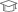Delhi University 1998

Master of Arts (M.A.)

Hauz Khas, Delhi, India - 110016

## Verified Info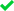ID VerifiedPhone VerifiedEmail Verified

Report this Profile

Is this listing inaccurate or duplicate? Any other problem?

Type the letters as shown below *

Please enter the letters as show below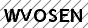Class 6 Tuition

Class Location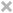Online (video chat via skype, google hangout etc)Student's HomeTutor's Home

Years of Experience in Class 6 Tuition

12

Board

CBSE

CBSE Subjects taught

Mathematics

Taught in School or College

No

## Reviews

this is test message this is test message this is test message this is test message this is test message this is test message this is test message

No Reviews yet! Be the first one to Review

## FAQs

1. Which school boards of Class 8 do you teach for?

CBSE

2. Have you ever taught in any School or College?

No

3. Which classes do you teach?

I teach Class 10 Tuition, Class 11 Tuition, Class 12 Tuition, Class 6 Tuition, Class 7 Tuition, Class 8 Tuition, Class 9 Tuition, Class I-V Tuition, Drawing and Vedic Maths Training Classes.

4. Do you provide a demo class?

Yes, I provide a paid demo class.

5. How many years of experience do you have?

I have been teaching for 12 years.

## Answers by Sumita D. (1)

Let the sides of first and second square be X and Y . Area of first square = (X)²And,Area of second square = (Y)²According to question,(X)² + (Y)² = 468 m² ------------(1).Perimeter of first square = 4 × Xand,Perimeter of second square = 4 × YAccording... ...more

Let the sides of first and second square be X and Y .

Area of first square = (X)²

And,

Area of second square = (Y)²

According to question,

(X)² + (Y)² = 468 m² ------------(1).

Perimeter of first square = 4 × X

and,

Perimeter of second square = 4 × Y

According to question,

4X - 4Y = 24 -----------(2)

From equation (2) we get,

4X - 4Y = 24

4(X-Y) = 24

X - Y = 24/4

X - Y = 6

X = 6+Y ---------(3)

Putting the value of X in equation (1)

(X)² + (Y)² = 468

(6+Y)² + (Y)² = 468

(6)² + (Y)² + 2 × 6 × Y + (Y)² = 468

36 + Y² + 12Y + Y² = 468

2Y² + 12Y - 468 +36 = 0

2Y² + 12Y -432 = 0

2( Y² + 6Y - 216) = 0

Y² + 6Y - 216 = 0

Y² + 18Y - 12Y -216 = 0

Y(Y+18) - 12(Y+18) = 0

(Y+18) (Y-12) = 0

(Y+18) = 0 Or (Y-12) = 0

Y = -18 OR Y = 12

Putting Y = 12 in EQUATION (3)

X = 6+Y = 6+12 = 18

Side of first square = X = 18 m

and,

Side of second square = Y = 12 m.
Dislike Bookmark
Class 6 Tuition 5.0

Class LocationOnline (video chat via skype, google hangout etc)Student's HomeTutor's Home

Years of Experience in Class 6 Tuition

12

Board

CBSE

CBSE Subjects taught

Mathematics

Taught in School or College

No

Class 7 Tuition 5.0

Class LocationOnline (video chat via skype, google hangout etc)Student's HomeTutor's Home

Years of Experience in Class 7 Tuition

8

Board

ICSE, CBSE

CBSE Subjects taught

Science, Mathematics

ICSE Subjects taught

Mathematics

Taught in School or College

No

Class 8 Tuition 5.0

Class LocationOnline (video chat via skype, google hangout etc)Student's HomeTutor's Home

Years of Experience in Class 8 Tuition

8

Board

ICSE, CBSE

CBSE Subjects taught

Science, Mathematics

ICSE Subjects taught

Mathematics

Taught in School or College

No

Class 9 Tuition 5.0

Class LocationOnline (video chat via skype, google hangout etc)Student's HomeTutor's Home

Years of Experience in Class 9 Tuition

10

Board

CBSE

CBSE Subjects taught

Mathematics

Taught in School or College

Yes

Class 10 Tuition 5.0

Class LocationOnline (video chat via skype, google hangout etc)Student's HomeTutor's Home

Years of Experience in Class 10 Tuition

10

Board

CBSE

CBSE Subjects taught

Mathematics

Taught in School or College

No

Drawing Classes 5.0

Class LocationOnline (video chat via skype, google hangout etc)Student's HomeTutor's Home

Years of Experience in Drawing Classes

7

Class 11 Tuition 5.0

Class LocationOnline (video chat via skype, google hangout etc)Student's HomeTutor's Home

Years of Experience in Class 11 Tuition

8

Board

CBSE

CBSE Subjects taught

Mathematics

Taught in School or College

No

Class 12 Tuition 5.0

Class LocationOnline (video chat via skype, google hangout etc)Student's HomeTutor's Home

Years of Experience in Class 12 Tuition

8

Board

CBSE

CBSE Subjects taught

Mathematics, Accountancy, Economics

Taught in School or College

No

Vedic Maths Training classes 5.0

Class LocationOnline (video chat via skype, google hangout etc)Student's HomeTutor's Home

Years of Experience in Vedic Maths Training classes

12

Class I-V Tuition 5.0

Class LocationOnline (video chat via skype, google hangout etc)Student's HomeTutor's Home

Years of Experience in Class I-V Tuition

8

Board

State, International Baccalaureate, CBSE, ICSE

IB Subjects taught

Mathematics, Science

CBSE Subjects taught

Science, EVS, Mathematics

ICSE Subjects taught

Science, EVS, Mathematics

Taught in School or College

No

State Syllabus Subjects taught

EVS, Science, Mathematics

this is test message this is test message this is test message this is test message this is test message this is test message this is test message

No Reviews yet! Be the first one to Review

## Answers by Sumita D. (1)

Let the sides of first and second square be X and Y . Area of first square = (X)²And,Area of second square = (Y)²According to question,(X)² + (Y)² = 468 m² ------------(1).Perimeter of first square = 4 × Xand,Perimeter of second square = 4 × YAccording... ...more

Let the sides of first and second square be X and Y .

Area of first square = (X)²

And,

Area of second square = (Y)²

According to question,

(X)² + (Y)² = 468 m² ------------(1).

Perimeter of first square = 4 × X

and,

Perimeter of second square = 4 × Y

According to question,

4X - 4Y = 24 -----------(2)

From equation (2) we get,

4X - 4Y = 24

4(X-Y) = 24

X - Y = 24/4

X - Y = 6

X = 6+Y ---------(3)

Putting the value of X in equation (1)

(X)² + (Y)² = 468

(6+Y)² + (Y)² = 468

(6)² + (Y)² + 2 × 6 × Y + (Y)² = 468

36 + Y² + 12Y + Y² = 468

2Y² + 12Y - 468 +36 = 0

2Y² + 12Y -432 = 0

2( Y² + 6Y - 216) = 0

Y² + 6Y - 216 = 0

Y² + 18Y - 12Y -216 = 0

Y(Y+18) - 12(Y+18) = 0

(Y+18) (Y-12) = 0

(Y+18) = 0 Or (Y-12) = 0

Y = -18 OR Y = 12

Putting Y = 12 in EQUATION (3)

X = 6+Y = 6+12 = 18

Side of first square = X = 18 m

and,

Side of second square = Y = 12 m.
Dislike Bookmark

Sumita D. describes herself as Tutor. She conducts classes in Class 10 Tuition, Class 11 Tuition and Class 12 Tuition. Sumita is located in Hauz Khas, Delhi. Sumita takes at students Home. She has 12 years of teaching experience . Sumita has completed Master of Arts (M.A.) from Delhi University in 1998. She is well versed in Hindi and English.

•• Want to learn from Sumita D.?

• Contact Now
X

Recommended Profiles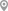Uttam Nagar, DelhiAshram, DelhiBegumpur, DelhiShakarpur, DelhiMalad East, MumbaiMogappair East, Chennai

X

Let us shortlist and give the best tutors and institutes.

or

Send Enquiry to Sumita D.

Let Sumita D. know you are interested in their class